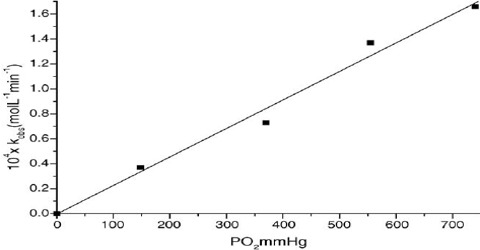Chemistry

# Dependence of Rate on Concentration: Explanation with ExampleDependence of Rate on Concentration

The type of dependence, which is always determined by experiment, is peculiar to a particular reaction. Some examples are:

(a) In the reaction 2 N2O5 (g) → 4NO2 (g) + O2 (g)

The rate is directly proportional to the concentration of N2O5 (g). So, the rate equation for this reaction is; rate = k [N2O5]

In this expression the value of n is one. So it is called a first order reaction.

(b) In the reaction N2O5Br (aq) + OH (aq) → N2O5OH (aq) + Br (aq)

The rate is proportional to the concentration of C2H5Br and of OH. So the rate equation is:

rate = k [C2H5Br] [OH]

In this reaction the order with respect to C2H5Br is one and the order with respect to OH is also one. The overall order is (1 + 1 = 2) two.

(c) In the reaction (CH3)3CBr (aq) + OH (aq) → (CH3)3COH (aq) + Br (aq)

The rate is directly proportional only to the concentration of (CH3)3CBr. So the rate equation is:

rate = k [(CH3)3CBr]

and the order of the reaction is one. Since the rate does not depend on concentration of OH the order with respect to OH ion is said to be zero.

(d) In the reaction 2NO (g) + 2H2 (g) = N2 (g) + 2H2O (g)

the rate is proportional to the concentration of H2 (g) and to the square of the concentration of NO (g). The rate equation fix this reaction is:

rate = k [NO]2 [H2]

In this reaction order with respect to NO is two and order with respect to H2 is one and the overall order is (1 + 2 = 3) three.

There are some reactions where the rate is not dependent on the concentration of a reactant as in (c) above in which the rate is independent of the concentration of OH. Such reactions are known as zero order reaction. One also finds reactions of fractional order like ½, 1/3, ¼ etc.

It is clear from the above examples that the order of a reaction is an experimental quantity and does not depend on the co-efficients of the reactants in the balanced chemical equation for the reaction.